SEARCH HOMEMath Central Quandaries & Querieslynda, a student: (-4x)to the 0 power times x squared over 3to the -3 power i have to rewrite the expression with pos exponentsHi Lynda,

Here are the exponent laws:

 Product Law Quotient Law Power Law xa·xb=xa+b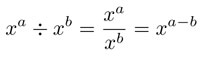(xa)b=xab Zero Power Law Power of a Product Law Power of a Quotient Law x0=1 (xy)a=xaya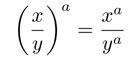Negative Exponent Law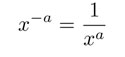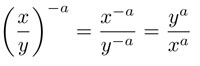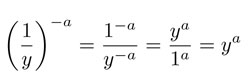Rational Exponent Law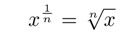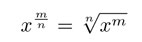Let's look at a similar example to your problem: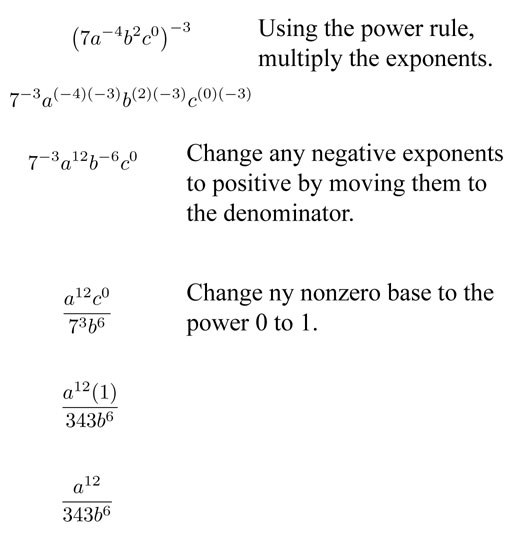Hope this helps,

JaniceMath Central is supported by the University of Regina and The Pacific Institute for the Mathematical Sciences.